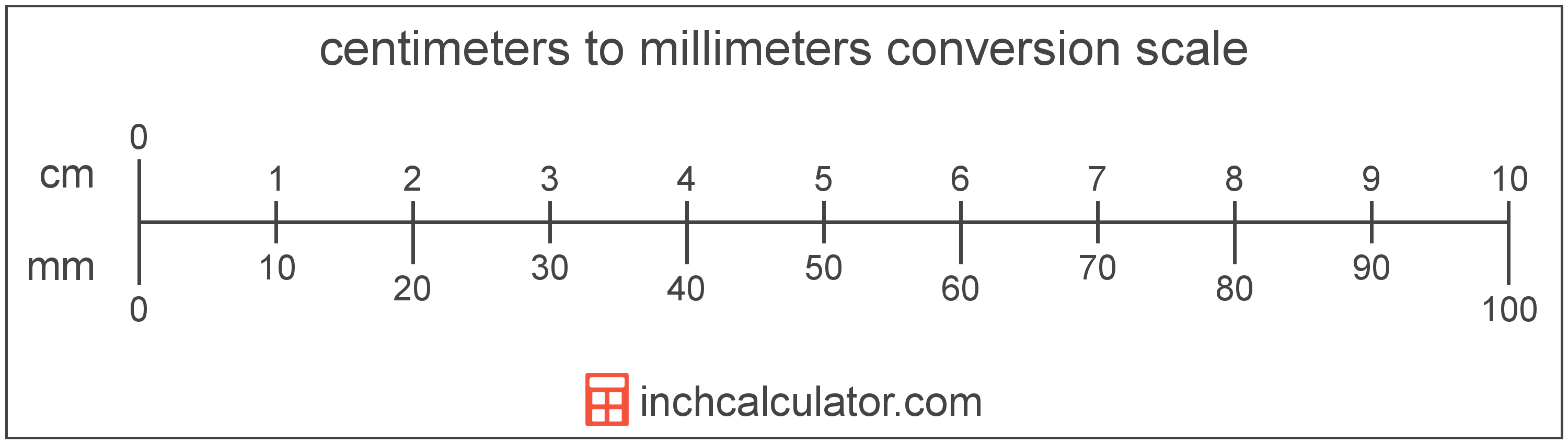# Millimeters to Centimeters Converter

Enter the length in millimeters below to get the value converted to centimeters.

Results in Centimeters:1 mm = 0.1 cm

Do you want to convert centimeters to millimeters?

## How to Convert Millimeters to Centimeters

To convert a measurement in millimeters to a measurement in centimeters, divide the length by the following conversion ratio: 10 millimeters/centimeter.

Since one centimeter is equal to 10 millimeters, you can use this simple formula to convert:

centimeters = millimeters ÷ 10

The length in centimeters is equal to the length in millimeters divided by 10.

For example, here's how to convert 5 millimeters to centimeters using the formula above.
centimeters = (5 mm ÷ 10) = 0.5 cmOur inch fraction calculator can add millimeters and centimeters together, and it also automatically converts the results to US customary, imperial, and SI metric values.

## What Is a Millimeter?

One millimeter is equal to one-thousandth (1/1,000) of a meter, which is defined as the distance light travels in a vacuum in 1/299,792,458 of a second. One millimeter is equal to 0.0393701 inches.

The millimeter, or millimetre, is a multiple of the meter, which is the SI base unit for length. In the metric system, "milli" is the prefix for thousandths, or 10-3. Millimeters can be abbreviated as mm; for example, 1 millimeter can be written as 1 mm.

Millimeters are often represented by the smallest ticks on most metric rulers. To get a reference point of the size, the thickness of a US dime is 1.35mm.

## What Is a Centimeter?

One centimeter is equal to one-hundredth (1/100) of a meter, which is defined as the distance light travels in a vacuum in 1/299,792,458 of a second. One centimeter is equal to 0.393701 inches.

The centimeter, or centimetre, is a multiple of the meter, which is the SI base unit for length. In the metric system, "centi" is the prefix for hundredths, or 10-2. Centimeters can be abbreviated as cm; for example, 1 centimeter can be written as 1 cm.

Metric rulers typically have 30 cm, which are represented by 30 large tick marks. To get a rough idea of the actual length of a centimeter, a standard number 2 pencil is just about 1 cm thick.

We recommend using a ruler or tape measure for measuring length, which can be found at a local retailer or home center. Rulers are available in imperial, metric, or a combination of both values, so make sure you get the correct type for your needs.

Need a ruler? Try our free downloadable and printable rulers, which include both imperial and metric measurements.

## Millimeter to Centimeter Conversion Table

Table showing various millimeter measurements converted to centimeters.
Millimeters Centimeters
1 mm 0.1 cm
2 mm 0.2 cm
3 mm 0.3 cm
4 mm 0.4 cm
5 mm 0.5 cm
6 mm 0.6 cm
7 mm 0.7 cm
8 mm 0.8 cm
9 mm 0.9 cm
10 mm 1 cm

## References

1. United States Mint, Coin Specifications, https://www.usmint.gov/learn/coin-and-medal-programs/coin-specifications